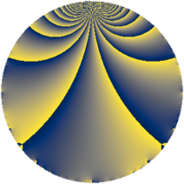# Properties

 Label 775.2.bLevel $775$ Weight $2$ Character orbit 775.b Rep. character $\chi_{775}(249,\cdot)$ Character field $\Q$ Dimension $46$ Newform subspaces $8$ Sturm bound $160$ Trace bound $9$

# Related objects

## Defining parameters

 Level: $$N$$ $$=$$ $$775 = 5^{2} \cdot 31$$ Weight: $$k$$ $$=$$ $$2$$ Character orbit: $$[\chi]$$ $$=$$ 775.b (of order $$2$$ and degree $$1$$) Character conductor: $$\operatorname{cond}(\chi)$$ $$=$$ $$5$$ Character field: $$\Q$$ Newform subspaces: $$8$$ Sturm bound: $$160$$ Trace bound: $$9$$ Distinguishing $$T_p$$: $$2$$

## Dimensions

The following table gives the dimensions of various subspaces of $$M_{2}(775, [\chi])$$.

Total New Old
Modular forms 86 46 40
Cusp forms 74 46 28
Eisenstein series 12 0 12

## Trace form

 $$46 q - 48 q^{4} - 8 q^{6} - 34 q^{9} + O(q^{10})$$ $$46 q - 48 q^{4} - 8 q^{6} - 34 q^{9} - 12 q^{11} + 2 q^{14} + 68 q^{16} - 8 q^{19} - 28 q^{21} + 28 q^{24} - 28 q^{26} + 16 q^{29} + 6 q^{31} - 52 q^{34} - 28 q^{36} - 4 q^{39} + 60 q^{44} + 20 q^{46} - 10 q^{49} - 36 q^{51} - 24 q^{59} + 12 q^{61} - 110 q^{64} - 4 q^{66} + 32 q^{69} - 16 q^{71} - 12 q^{74} - 22 q^{76} + 64 q^{79} + 22 q^{81} + 76 q^{84} - 64 q^{86} + 44 q^{89} - 72 q^{91} + 32 q^{94} - 52 q^{96} + 136 q^{99} + O(q^{100})$$

## Decomposition of $$S_{2}^{\mathrm{new}}(775, [\chi])$$ into newform subspaces

Label Dim $A$ Field CM Traces $q$-expansion
$a_{2}$ $a_{3}$ $a_{5}$ $a_{7}$
775.2.b.a $2$ $6.188$ $$\Q(\sqrt{-1})$$ None $$0$$ $$0$$ $$0$$ $$0$$ $$q+2iq^{2}-iq^{3}-2q^{4}+2q^{6}+2iq^{7}+\cdots$$
775.2.b.b $2$ $6.188$ $$\Q(\sqrt{-1})$$ None $$0$$ $$0$$ $$0$$ $$0$$ $$q+iq^{2}+2iq^{3}+q^{4}-2q^{6}-4iq^{7}+\cdots$$
775.2.b.c $2$ $6.188$ $$\Q(\sqrt{-1})$$ None $$0$$ $$0$$ $$0$$ $$0$$ $$q-iq^{3}+2q^{4}+2q^{9}-4q^{11}-2iq^{12}+\cdots$$
775.2.b.d $4$ $6.188$ $$\Q(i, \sqrt{5})$$ None $$0$$ $$0$$ $$0$$ $$0$$ $$q+\beta _{1}q^{2}+2\beta _{1}q^{3}+(1+\beta _{2})q^{4}+(-2+\cdots)q^{6}+\cdots$$
775.2.b.e $8$ $6.188$ $$\mathbb{Q}[x]/(x^{8} - \cdots)$$ None $$0$$ $$0$$ $$0$$ $$0$$ $$q-\beta _{7}q^{2}-\beta _{4}q^{3}+(-2+\beta _{3}+\beta _{6}+\cdots)q^{4}+\cdots$$
775.2.b.f $8$ $6.188$ 8.0.4589249536.2 None $$0$$ $$0$$ $$0$$ $$0$$ $$q+\beta _{5}q^{2}-\beta _{1}q^{3}+(-1-\beta _{4})q^{4}+(-1+\cdots)q^{6}+\cdots$$
775.2.b.g $10$ $6.188$ $$\mathbb{Q}[x]/(x^{10} + \cdots)$$ None $$0$$ $$0$$ $$0$$ $$0$$ $$q+(\beta _{4}+\beta _{7})q^{2}+(-\beta _{4}-\beta _{8})q^{3}+(-1+\cdots)q^{4}+\cdots$$
775.2.b.h $10$ $6.188$ $$\mathbb{Q}[x]/(x^{10} + \cdots)$$ None $$0$$ $$0$$ $$0$$ $$0$$ $$q+(\beta _{1}+\beta _{2})q^{2}+\beta _{3}q^{3}+(-2+\beta _{6}+\cdots)q^{4}+\cdots$$

## Decomposition of $$S_{2}^{\mathrm{old}}(775, [\chi])$$ into lower level spaces

$$S_{2}^{\mathrm{old}}(775, [\chi]) \cong$$ $$S_{2}^{\mathrm{new}}(155, [\chi])$$$$^{\oplus 2}$$